Next: Other Methods Up: Soft Competitive Learning with Previous: Growing Cell Structures

# Growing Grid

Growing grid is another incremental network. The basic principles used also in growing cell structures and growing neural gas are applied with some modifications to a rectangular grid. Alternatively, growing grid can be seen as an incremental variant of the self-organizing feature map.

The model has two distinct phases, a growth phase and a fine-tuning phase. During the growth phase a rectangular network is built up starting from a minimal size by inserting complete rows and columns until the desired size is reached or until a performance criterion is met. Only constant parameters are used in this phase. In the fine-tuning phase the size of the network is not changed anymore and a decaying learning rate is used to find good final values for the reference vectors.

As for the self-organizing map, the network structure is a two-dimensional grid (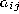). This grid is initially set tostructure. Again, the distance on the grid is used to determine how strongly a unitis adapted when the unit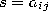is the winner. The distance measure used is the-norm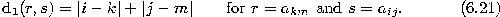Also the function used to determine the adaptation strength for a unit r given that s is the winner is the same as for the self-organizing feature map: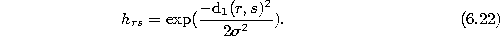The width parameter, however, remains constant throughout the whole simulation. It is chosen relatively small compared to the values usually used at the beginning for the self-organizing feature map. One can note that as the growing grid network grows, the fraction of all units which is adapted together with the winner decreases. This is also the case in the self-organizing feature map but is achieved there with a constant network size and a decreasing neighborhood width. The complete growing grid algorithm is the following:

Growth Phase

1. Set the initial network width and height:Initialize the set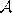to contain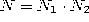units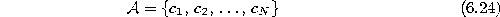with reference vectorschosen randomly according to.

Initialize the connection setto form a rectangular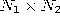grid.

Initialize the time parameter t:2. Generate at random an input signalaccording to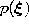.
3. Determine the winner:4. Increase a local counter variable of the winner:5. Increase the time parameter t: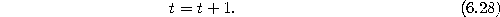6. Adapt each unit r according towhereby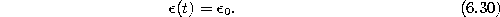7. If the number of input signals generated for the current network size reaches a multipleof this network size, i.e., if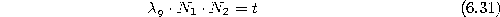then do the following:
• Determine the unit q with the largest value of: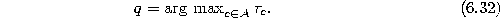• Determine the direct neighbor f of q with the most distant reference vector: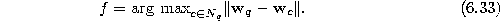• Depending on the relative position of q and f continue with one of the two following cases:
Case 1: q and f are in the same row of the grid, i.e.Do the following:
• Insert a new column withunits between the columns of q and f.
• Interpolate the reference vectors of the new units from the reference vectors of their respective direct neigbors in the same row.
• Adjust the variable for the number of columns: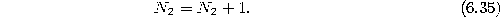Case 2: q and f are in the same column of the grid, i.e.Do the following:
• Insert a new row withunits between the rows of q and f.
• Interpolate the reference vectors of the new units from the reference vectors of their respective direct neigbors in the same columns.
• Adjust the variable for the number of rows:• reset all local counter values: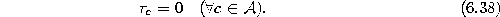• reset the time parameter:8. If the desired network size is not yet achieved, i.e. if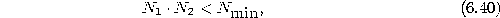then continue with step 2.

Fine-tuning Phase

9.
Generate at random an input signal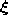according to.
10.
Determine the winner:11.
Adapt each unit r according towherebywith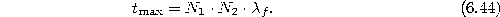12.
If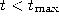continue with step 9.

Figure 6.5 shows some stages of a simulation for a simple ring-shaped data distribution. Figure 6.6 displays the final results after 40000 adaptation steps for three other distribution. The parameters used for the growth phase were:. The parameters for the fine-tuning phase were:andunchanged,.Figure 6.5:   Growing grid simulation sequence for a ring-shaped uniform probability distribution. a) Initial state. b-f) Intermediate states. g) Final state. h) Voronoi tessellation corresponding to the final state.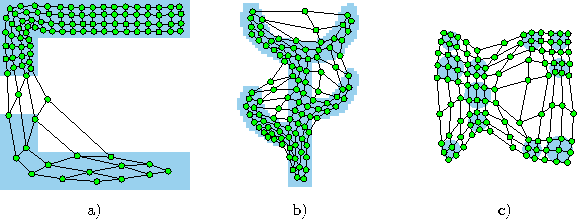Figure:   Growing grid simulation results after 40000 input signals for three different probability distributions (described in the caption of figure 4.4). One can note that in a) the chosen topology () has a rather extreme height/width ratio which matches well the distribution at hand. Depending on initial conditions however, also other topologies occur in simulations for this distribution. b),c) Also these topologies deviate from the square shape usually given to self-organizing maps. For the cactus a (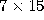) and for the mixture distribution a (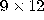) topology was automatically selected by the algorithm.

If one compares the growing grid algorithm with the other incremental methods growing cell structures and growing neural gas then a difference (apart from the topology) is that no counter variables are redistributed when new units are inserted. Instead, all-values are set to zero after a row or column has been inserted. This means, that all statistical information about winning frequencies is discarded after an insertion. Therefore, to gather enough statistical evidence where to insert new units the next time, the number of adaptation steps per insertion step must be proportional to the network size (see equation 6.31). This simplifies the algorithm but increases the computational complexity. The same could in principle be done with growing neural gas and growing cell structures effectively eliminating the need to re-distribute accumulated information after insertions at the price of increased computational complexity.

The parameterwhich governs the neighborhood range has the function of a regularizer. If it is set to large values, then neighboring units are forced to have rather similar reference vectors and the layout of the network (when projected to input space) will appear very regular but not so well adapted to the underlying data distribution. Smaller values forgive the units more possibilities to adapt independently from each other. Asis set more and more to zero the growing grid algorithm (apart from the insertions) approaches hard competitive learning.

Similar to the self-organizing feature map the growing grid algorithm can easily be applied to network structures of other dimensions than two. Actually useful, however, seem only the cases of one- and three-dimensional networks since networks of higher dimensionality can not be visualized easily.Next: Other Methods Up: Soft Competitive Learning with Previous: Growing Cell Structures

Bernd Fritzke
Sat Apr 5 18:17:58 MET DST 1997